## 一篇写数据时间序列分析的不错的文章 转

o
osc_fmg49rzg

https://www.cnblogs.com/foley/p/5582358.html#undefined

时间序列简单的说就是各时间点上形成的数值序列，时间序列分析就是通过观察历史数据预测未来的值。在这里需要强调一点的是，时间序列分析并不是关于时间的回归，它主要是研究自身的变化规律的（这里不考虑含外生变量的时间序列）。

两个字总结“情怀”，爱屋及乌，个人比较喜欢python，就用python撸了。能做时间序列的软件很多，SAS、R、SPSS、Eviews甚至matlab等等，实际工作中应用得比较多的应该还是SAS和R，前者推荐王燕写的《应用时间序列分析》，后者推荐“基于R语言的时间序列建模完整教程”这篇博文（翻译版）。python作为科学计算的利器，当然也有相关分析的包:statsmodels中tsa模块，当然这个包和SAS、R是比不了，但是python有另一个神器：pandas！pandas在时间序列上的应用，能简化我们很多的工作。

python推荐直接装Anaconda，它集成了许多科学计算包，有一些包自己手动去装还是挺费劲的。statsmodels需要自己去安装，这里我推荐使用0.6的稳定版，0.7及其以上的版本能在github上找到，该版本在安装时会用C编译好，所以修改底层的一些代码将不会起作用。

1.基本模型

自回归移动平均模型(ARMA(p，q))是时间序列中最为重要的模型之一，它主要由两部分组成： AR代表p阶自回归过程，MA代表q阶移动平均过程，其公式如下：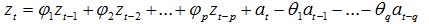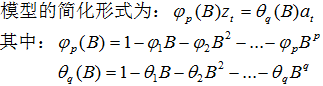依据模型的形式、特性及自相关和偏自相关函数的特征，总结如下：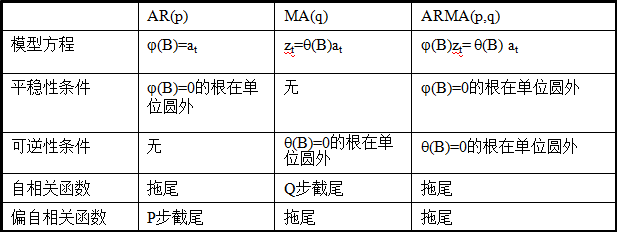2.pandas时间序列操作

``````# -*- coding:utf-8 -*-
import numpy as np
import pandas as pdfrom datetime import datetimeimport matplotlib.pylab as plt``````
``# 读取数据，pd.read_csv默认生成DataFrame对象，需将其转换成Series对象df = pd.read_csv('AirPassengers.csv', encoding='utf-8', index_col='date')df.index = pd.to_datetime(df.index)  # 将字符串索引转换成时间索引ts = df['x']  # 生成pd.Series对象# 查看数据格式ts.head()ts.head().index``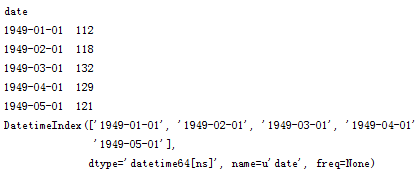``````ts['1949-01-01']
ts[datetime(1949,1,1)]``````

``ts['1949']````ts['1949-1' : '1949-6']``pandas还有很多方便的时间序列函数，在后面的实际应用中在进行说明。

3. 平稳性检验• 常数均值
• 常数方差
• 常数自协方差

``````# -*- coding:utf-8 -*-
from statsmodels.tsa.stattools import adfuller import pandas as pd import matplotlib.pyplot as plt import numpy as np from statsmodels.graphics.tsaplots import plot_acf, plot_pacf # 移动平均图 def draw_trend(timeSeries, size): f = plt.figure(facecolor='white') # 对size个数据进行移动平均 rol_mean = timeSeries.rolling(window=size).mean() # 对size个数据进行加权移动平均 rol_weighted_mean = pd.ewma(timeSeries, span=size) timeSeries.plot(color='blue', label='Original') rolmean.plot(color='red', label='Rolling Mean') rol_weighted_mean.plot(color='black', label='Weighted Rolling Mean') plt.legend(loc='best') plt.title('Rolling Mean') plt.show() def draw_ts(timeSeries): f = plt.figure(facecolor='white') timeSeries.plot(color='blue') plt.show() '''　　Unit Root Test The null hypothesis of the Augmented Dickey-Fuller is that there is a unit root, with the alternative that there is no unit root. That is to say the bigger the p-value the more reason we assert that there is a unit root ''' def testStationarity(ts): dftest = adfuller(ts) # 对上述函数求得的值进行语义描述 dfoutput = pd.Series(dftest[0:4], index=['Test Statistic','p-value','#Lags Used','Number of Observations Used']) for key,value in dftest.items(): dfoutput['Critical Value (%s)'%key] = value return dfoutput # 自相关和偏相关图，默认阶数为31阶 def draw_acf_pacf(ts, lags=31): f = plt.figure(facecolor='white') ax1 = f.add_subplot(211) plot_acf(ts, lags=31, ax=ax1) ax2 = f.add_subplot(212) plot_pacf(ts, lags=31, ax=ax2) plt.show()``````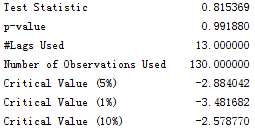3. 平稳性处理

a. 对数变换

``````ts_log = np.log(ts)
test_stationarity.draw_ts(ts_log)``````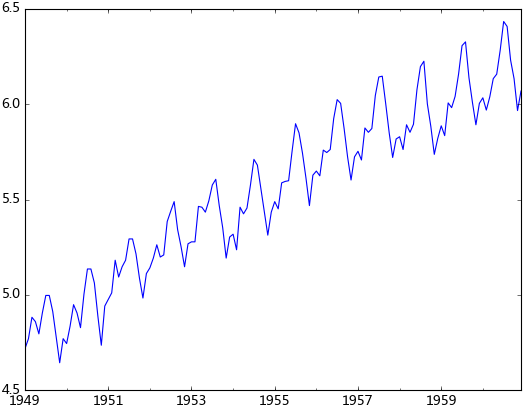b. 平滑法

``test_stationarity.draw_trend(ts_log, 12)``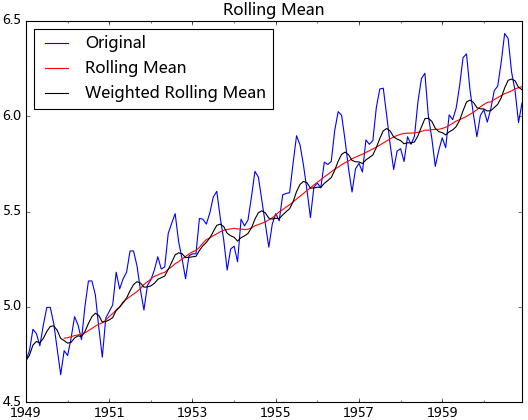c.  差分``````diff_12 = ts_log.diff(12)
diff_12.dropna(inplace=True)
diff_12_1 = diff_12.diff(1)
diff_12_1.dropna(inplace=True)
test_stationarity.testStationarity(diff_12_1)``````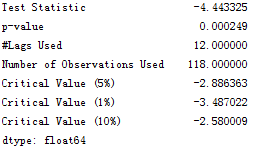d. 分解

``````from statsmodels.tsa.seasonal import seasonal_decompose
decomposition = seasonal_decompose(ts_log, model="additive") trend = decomposition.trend seasonal = decomposition.seasonal residual = decomposition.resid``````4. 模型识别

``````rol_mean = ts_log.rolling(window=12).mean()
rol_mean.dropna(inplace=True)
ts_diff_1 = rol_mean.diff(1)
ts_diff_1.dropna(inplace=True)
test_stationarity.testStationarity(ts_diff_1)````````````ts_diff_2 = ts_diff_1.diff(1)
ts_diff_2.dropna(inplace=True)``````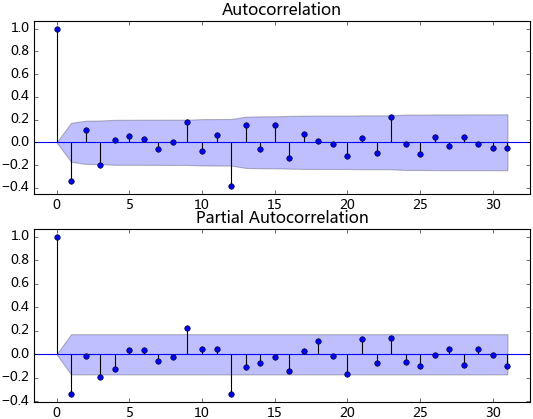``````from statsmodels.tsa.arima_model import ARMA
model = ARMA(ts_diff_2, order=(1, 1))
result_arma = model.fit( disp=-1, method='css')``````

5. 样本拟合

模型拟合完后，我们就可以对其进行预测了。由于ARMA拟合的是经过相关预处理后的数据，故其预测值需要通过相关逆变换进行还原。

``````predict_ts = result_arma.predict()
# 一阶差分还原diff_shift_ts = ts_diff_1.shift(1)diff_recover_1 = predict_ts.add(diff_shift_ts)# 再次一阶差分还原
rol_shift_ts = rol_mean.shift(1) diff_recover = diff_recover_1.add(rol_shift_ts) # 移动平均还原 rol_sum = ts_log.rolling(window=11).sum() rol_recover = diff_recover*12 - rol_sum.shift(1) # 对数还原 log_recover = np.exp(rol_recover) log_recover.dropna(inplace=True)``````

``````ts = ts[log_recover.index]  # 过滤没有预测的记录plt.figure(facecolor='white')
log_recover.plot(color='blue', label='Predict') ts.plot(color='red', label='Original') plt.legend(loc='best') plt.title('RMSE: %.4f'% np.sqrt(sum((log_recover-ts)**2)/ts.size)) plt.show()``````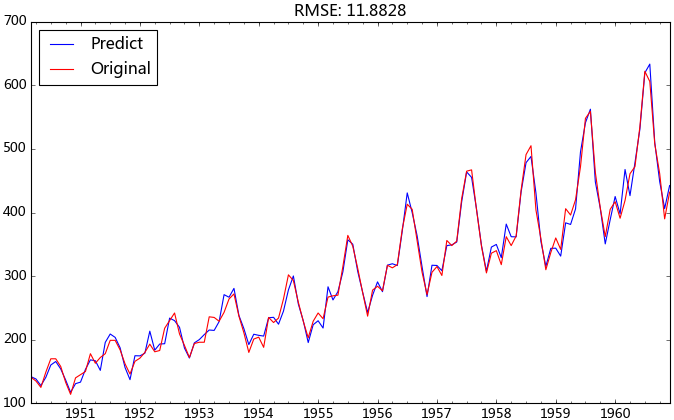6. 完善ARIMA模型

``````# 差分操作
def diff_ts(ts, d):
global shift_ts_list # 动态预测第二日的值时所需要的差分序列 global last_data_shift_list shift_ts_list = [] last_data_shift_list = [] tmp_ts = ts for i in d: last_data_shift_list.append(tmp_ts[-i]) print last_data_shift_list shift_ts = tmp_ts.shift(i) shift_ts_list.append(shift_ts) tmp_ts = tmp_ts - shift_ts tmp_ts.dropna(inplace=True) return tmp_ts # 还原操作 def predict_diff_recover(predict_value, d): if isinstance(predict_value, float): tmp_data = predict_value for i in range(len(d)): tmp_data = tmp_data + last_data_shift_list[-i-1] elif isinstance(predict_value, np.ndarray): tmp_data = predict_value for i in range(len(d)): tmp_data = tmp_data + last_data_shift_list[-i-1] else: tmp_data = predict_value for i in range(len(d)): try: tmp_data = tmp_data.add(shift_ts_list[-i-1]) except: raise ValueError('What you input is not pd.Series type!') tmp_data.dropna(inplace=True) return tmp_data``````

``````diffed_ts = diff_ts(ts_log, d=[12, 1])
model = arima_model(diffed_ts)
model.certain_model(1, 1)
predict_ts = model.properModel.predict()
diff_recover_ts = predict_diff_recover(predict_ts, d=[12, 1]) log_recover = np.exp(diff_recover_ts)````````````def proper_model(data_ts, maxLag):
init_bic = sys.maxint
init_p = 0
init_q = 0 init_properModel = None for p in np.arange(maxLag): for q in np.arange(maxLag): model = ARMA(data_ts, order=(p, q)) try: results_ARMA = model.fit(disp=-1, method='css') except: continue bic = results_ARMA.bic if bic < init_bic: init_p = p init_q = q init_properModel = results_ARMA init_bic = bic return init_bic, init_p, init_q, init_properModel``````

7.滚动预测

``from dateutil.relativedelta import relativedeltadef _add_new_data(ts, dat, type='day'):``
``if type == 'day': new_index = ts.index[-1] + relativedelta(days=1) elif type == 'month': new_index = ts.index[-1] + relativedelta(months=1) ts[new_index] = dat def add_today_data(model, ts, data, d, type='day'): _add_new_data(ts, data, type) # 为原始序列添加数据 # 为滞后序列添加新值 d_ts = diff_ts(ts, d) model.add_today_data(d_ts[-1], type) def forecast_next_day_data(model, type='day'): if model == None: raise ValueError('No model fit before') fc = model.forecast_next_day_value(type) return predict_diff_recover(fc, [12, 1])``

``````ts_train = ts_log[:'1956-12']
ts_test = ts_log['1957-1':] diffed_ts = diff_ts(ts_train, [12, 1]) forecast_list = [] for i, dta in enumerate(ts_test): if i%7 == 0: model = arima_model(diffed_ts) model.certain_model(1, 1) forecast_data = forecast_next_day_data(model, type='month') forecast_list.append(forecast_data) add_today_data(model, ts_train, dta, [12, 1], type='month') predict_ts = pd.Series(data=forecast_list, index=ts['1957-1':].index)log_recover = np.exp(predict_ts)original_ts = ts['1957-1':]``````8. 模型序列化

o

### osc_fmg49rzg#### 暂无文章

pycurl libcurl link-time ssl backend (nss)

pip uninstall pycurlecho 'pycurl==7.19.5.1 --global-option="--with-nss"' > requires.pypip install -r requires.py...

38分钟前
17
0

1、速率 单位时间(s)内传输信息(bit)量 单位：KB/s, MB/s, Gb/s K = 10^3 ,M = 10^6, G=10^9 一般表示的是理想的传输速率 2、带宽 计算机网络中的带宽和通信等领域的带宽概念不一样，计算机网...

osc_np3y0rbq
38分钟前
3
0

osc_5cok9i01
40分钟前
9
0

osc_j34n26zn
41分钟前
11
0

osc_0qnrwmy3
42分钟前
7
0